# A Complete Guide to Scatter Plots

Posted by Mike Yi

## What is a scatter plot?

A scatter plot (aka scatter chart, scatter graph) uses dots to represent values for two different numeric variables. The position of each dot on the horizontal and vertical axis indicates values for an individual data point. Scatter plots are used to observe relationships between variables.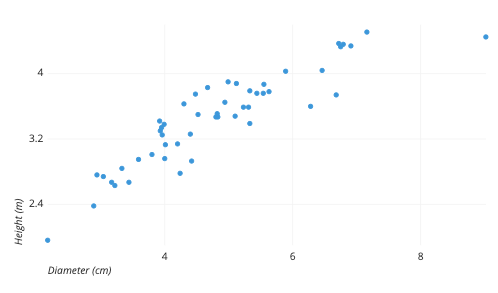The example scatter plot above shows the diameters and heights for a sample of fictional trees. Each dot represents a single tree; each point’s horizontal position indicates that tree’s diameter (in centimeters) and the vertical position indicates that tree’s height (in meters). From the plot, we can see a generally tight positive correlation between a tree’s diameter and its height. We can also observe an outlier point, a tree that has a much larger diameter than the others. This tree appears fairly short for its girth, which might warrant further investigation.

## When you should use a scatter plot

Scatter plots’ primary uses are to observe and show relationships between two numeric variables. The dots in a scatter plot not only report the values of individual data points, but also patterns when the data are taken as a whole.

Identification of correlational relationships are common with scatter plots. In these cases, we want to know, if we were given a particular horizontal value, what a good prediction would be for the vertical value. You will often see the variable on the horizontal axis denoted an independent variable, and the variable on the vertical axis the dependent variable. Relationships between variables can be described in many ways: positive or negative, strong or weak, linear or nonlinear.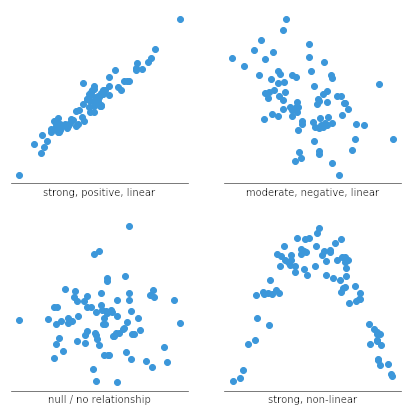A scatter plot can also be useful for identifying other patterns in data. We can divide data points into groups based on how closely sets of points cluster together. Scatter plots can also show if there are any unexpected gaps in the data and if there are any outlier points. This can be useful if we want to segment the data into different parts, like in the development of user personas.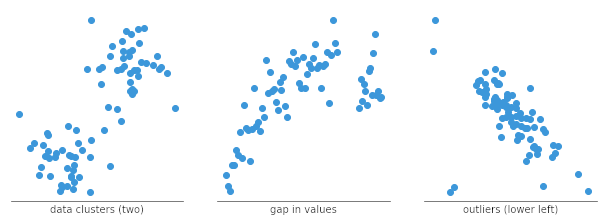### Example of data structure

diameter height
4.20 3.14
5.55 3.87
3.33 2.84
6.91 4.34

In order to create a scatter plot, we need to select two columns from a data table, one for each dimension of the plot. Each row of the table will become a single dot in the plot with position according to the column values.

## Common issues when using scatter plots

#### Overplotting

When we have lots of data points to plot, this can run into the issue of overplotting. Overplotting is the case where data points overlap to a degree where we have difficulty seeing relationships between points and variables. It can be difficult to tell how densely-packed data points are when many of them are in a small area.

There are a few common ways to alleviate this issue. One alternative is to sample only a subset of data points: a random selection of points should still give the general idea of the patterns in the full data. We can also change the form of the dots, adding transparency to allow for overlaps to be visible, or reducing point size so that fewer overlaps occur. As a third option, we might even choose a different chart type like the heatmap, where color indicates the number of points in each bin. Heatmaps in this use case are also known as 2-d histograms.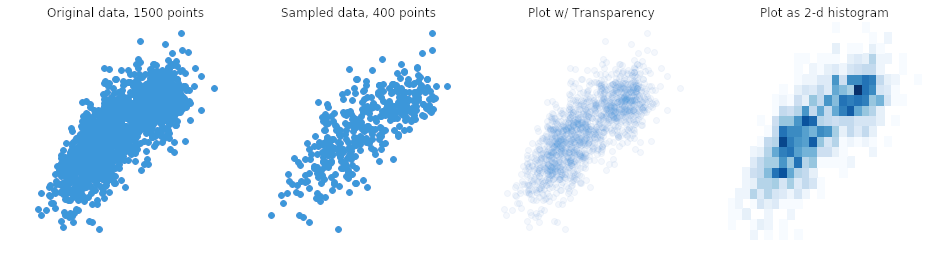#### Interpreting correlation as causation

This is not so much an issue with creating a scatter plot as it is an issue with its interpretation. Simply because we observe a relationship between two variables in a scatter plot, it does not mean that changes in one variable are responsible for changes in the other. This gives rise to the common phrase in statistics that correlation does not imply causation. It is possible that the observed relationship is driven by some third variable that affects both of the plotted variables, that the causal link is reversed, or that the pattern is simply coincidental.

For example, it would be wrong to look at city statistics for the amount of green space they have and the number of crimes committed and conclude that one causes the other, this can ignore the fact that larger cities with more people will tend to have more of both, and that they are simply correlated through that and other factors. If a causal link needs to be established, then further analysis to control or account for other potential variables effects needs to be performed, in order to rule out other possible explanations.

## Common scatter plot options

When a scatter plot is used to look at a predictive or correlational relationship between variables, it is common to add a trend line to the plot showing the mathematically best fit to the data. This can provide an additional signal as to how strong the relationship between the two variables is, and if there are any unusual points that are affecting the computation of the trend line.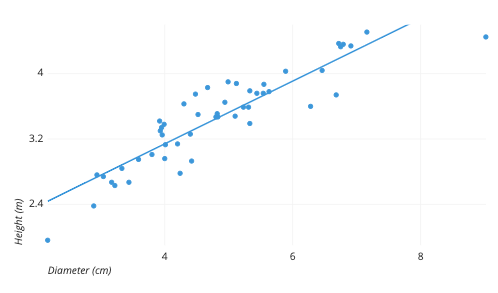#### Categorical third variable

A common modification of the basic scatter plot is the addition of a third variable. Values of the third variable can be encoded by modifying how the points are plotted. For a third variable that indicates categorical values (like geographical region or gender), the most common encoding is through point color. Giving each point a distinct hue makes it easy to show membership of each point to a respective group.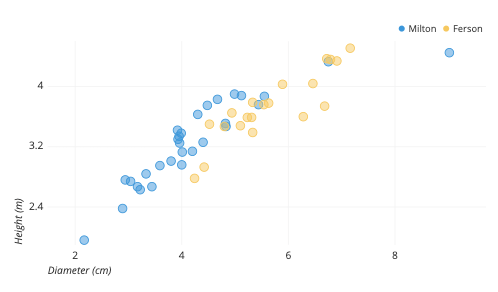Coloring points by tree type shows that Fersons (yellow) are generally wider than Miltons (blue), but also shorter for the same diameter.

One other option that is sometimes seen for third-variable encoding is that of shape. One potential issue with shape is that different shapes can have different sizes and surface areas, which can have an effect on how groups are perceived. However, in certain cases where color cannot be used (like in print), shape may be the best option for distinguishing between groups.The shapes above have been scaled to use the same amount of ink.

#### Numeric third variable

For third variables that have numeric values, a common encoding comes from changing the point size. A scatter plot with point size based on a third variable actually goes by a distinct name, the bubble chart. Larger points indicate higher values. A more detailed discussion of how bubble charts should be built can be read in its own article.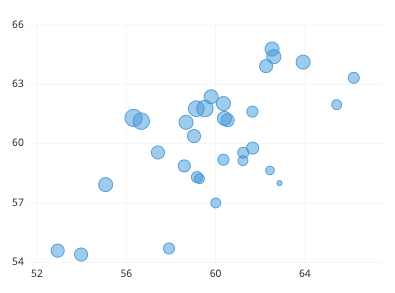Hue can also be used to depict numeric values as another alternative. Rather than using distinct colors for points like in the categorical case, we want to use a continuous sequence of colors, so that, for example, darker colors indicate higher value. Note that, for both size and color, a legend is important for interpretation of the third variable, since our eyes are much less able to discern size and color as easily as position.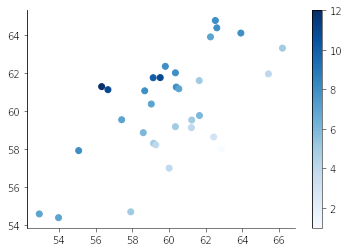#### Highlight using annotations and color

If you want to use a scatter plot to present insights, it can be good to highlight particular points of interest through the use of annotations and color. Desaturating unimportant points makes the remaining points stand out, and provides a reference to compare the remaining points against.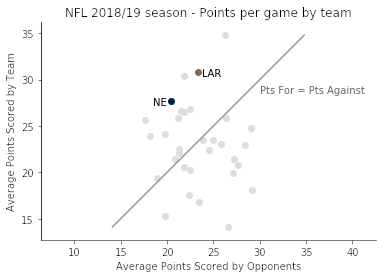#### Scatter map

When the two variables in a scatter plot are geographical coordinates – latitude and longitude – we can overlay the points on a map to get a scatter map (aka dot map). This can be convenient when the geographic context is useful for drawing particular insights and can be combined with other third-variable encodings like point size and color.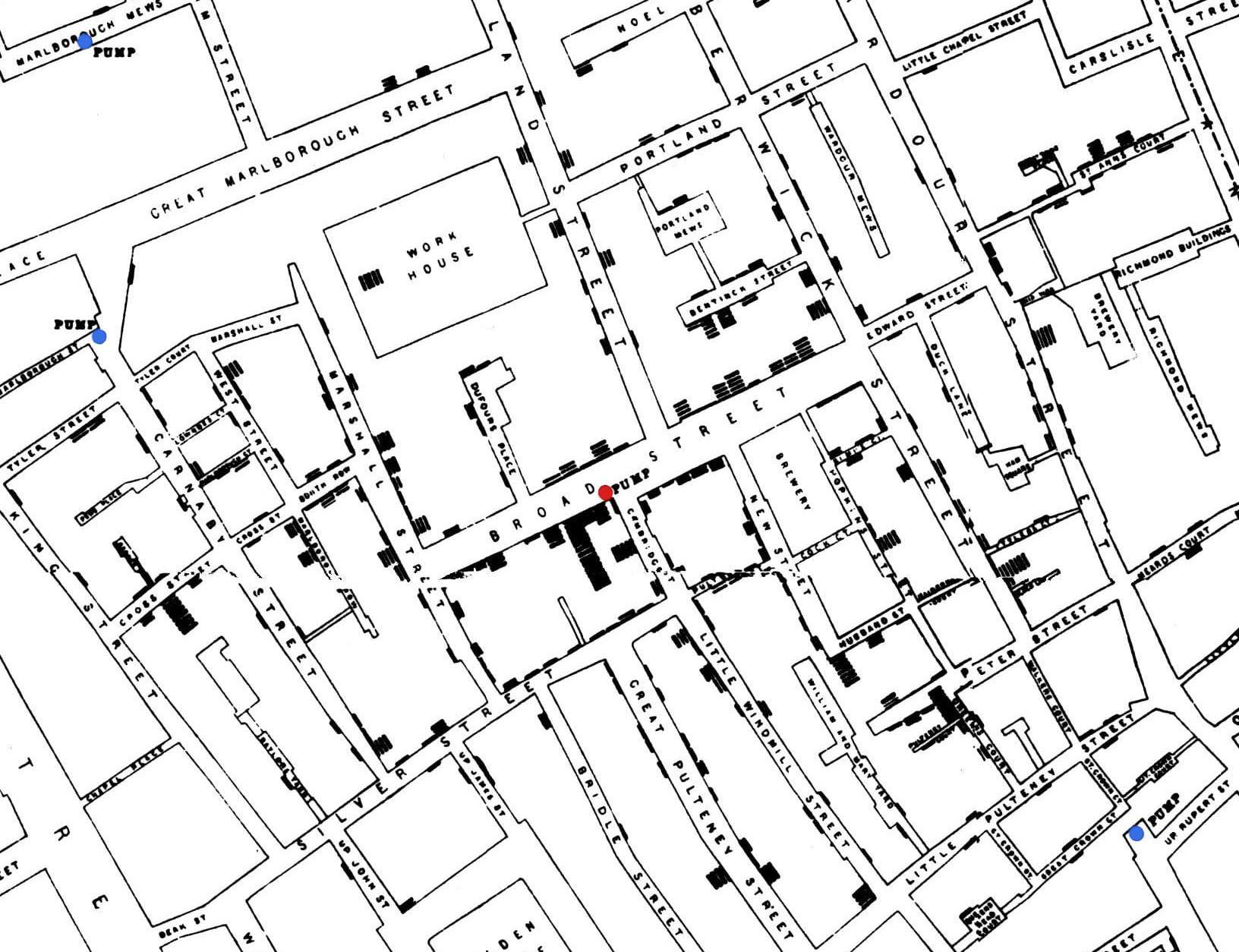A famous example of scatter map is John Snow’s 1854 cholera outbreak map, showing that cholera cases (black bars) were centered around a particular water pump on Broad Street (central dot). Original: Wikimedia Commons

#### Heatmap

As noted above, a heatmap can be a good alternative to the scatter plot when there are a lot of data points that need to be plotted and their density causes overplotting issues. However, the heatmap can also be used in a similar fashion to show relationships between variables when one or both variables are not continuous and numeric. If we try to depict discrete values with a scatter plot, all of the points of a single level will be in a straight line. Heatmaps can overcome this overplotting through their binning of values into boxes of counts.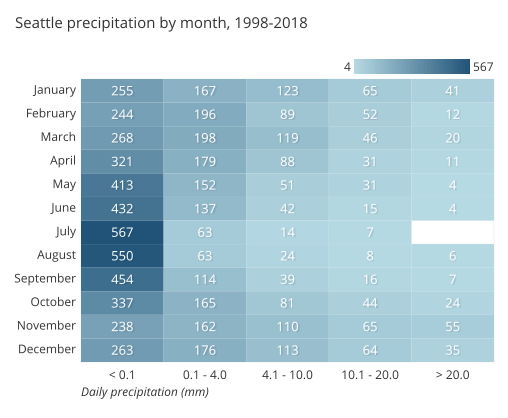#### Connected scatter plot

If the third variable we want to add to a scatter plot indicates timestamps, then one chart type we could choose is the connected scatter plot. Rather than modify the form of the points to indicate date, we use line segments to connect observations in order. This can make it easier to see how the two main variables not only relate to one another, but how that relationship changes over time. If the horizontal axis also corresponds with time, then all of the line segments will consistently connect points from left to right, and we have a basic line chart.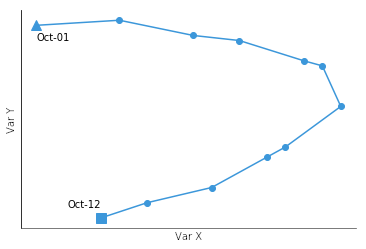## Visualization tools

The scatter plot is a basic chart type that should be creatable by any visualization tool or solution. Computation of a basic linear trend line is also a fairly common option, as is coloring points according to levels of a third, categorical variable. Other options, like non-linear trend lines and encoding third-variable values by shape, however, are not as commonly seen. Even without these options, however, the scatter plot can be a valuable chart type to use when you need to investigate the relationship between numeric variables in your data.

The scatter plot is one of many different chart types that can be used for visualizing data. Learn more from our articles on essential chart types, how to choose a type of data visualization, or by browsing the full collection of articles in the charts category.